Online Guide to MODFLOW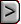HOB - Head-Observation Package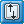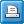HOB - Head-Observation PackageAbbreviation in Name file

HOB

Purpose

The Head-Observation input file is used to specify observations of head for use in the Observation process.

Documentation

 • Hill, M.C., Banta, E.R., Harbaugh, A.W., and Anderman, E.R., 2000, MODFLOW-2000, the U.S. Geological Survey modular ground-water model -- User guide to the Observation, Sensitivity, and Parameter-Estimation Processes and three post-processing programs: U.S. Geological Survey Open-File Report 00-184, 210 p.
 • release.txt
 • ofr-00-184-errata.pdf
 • OBS.pdf

Related Packages

Observation Process input files

Supported in

 • MODFLOW-2000
 • MODFLOW-2005
 • MODFLOW-LGR
 • MODFLOW-CFP
 • MODFLOW-NWT
 • MODFLOW-OWHM

Input Instructions

Input for the Head-Observation input file is read from a file that is specified with "HOB" as the file type in the name file.

Data Set 0

[#Text]

Item 0 is optional and can include as many lines as desired. Each line needs to begin with the “#” character in the first column.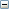Text
 Text—is a character string (maximum of 79 characters) that starts in column 2. Any characters can be included in Text. The “#” character needs to be in column 1. Text is printed when the file is read and provides an opportunity for the user to include information about the model both in the input file and the associated output file.

Data Set 1

 MODFLOW-2000: NH MOBS MAXM (free format) MODFLOW-2005: NH MOBS MAXM IUHOBSV HOBDRY [NOPRINT] (free format) MODFLOW-CFP, MODFLOW-NWT, MODFLOW-OWHM, and MODFLOW-LGR: NH MOBS MAXM IUHOBSV HOBDRY (free format)Explanation of variablesNHMOBS
 MOBS—is the number of the NH observations that are multilayer.MAXM
 MAXM—is the maximum number of layers used for any of the MOBS observations.IUHOBSV
 IUHOBSV—File unit for saving observation data in a file. Specify 0 for no observation output file. The file for this unit must be included as type “DATA” in the Name File.HOBDRY
 HOBDRY—is the value of the simulated equivalent that is written into the observation output file (file unit IUHOBSV) when the observation is omitted because a cell is dry.NOPRINT
 NOPRINT—is an option keyword that turns off printing of input and output data in the Listing File.

Data Set 2

 MODFLOW-2000: TOMULTH EVH (free format) MODFLOW-2005, MODFLOW-CFP, MODFLOW-NWT, MODFLOW-OWHM, and MODFLOW-LGR: TOMULTHExplanation of variablesTOMULTH
 TOMULTH—is the time-offset multiplier for head observations [-- or T/T]. The product of TOMULTH and TOFFSET must produce a time value in units consistent with other model input. TOMULTH can be dimensionless or can be used to convert the units of TOFFSET to the time unit used in the simulation.EVH
 EVH—is the input error variance multiplier for hydraulic-head observations and is used to calculate the weights as described below in the calculation of STATISTIC. EVH makes it easy to change the weights uniformly for all hydraulic-head observations.

Read sufficient repetitions of item 3 and, optionally, items 4 through 6 to obtain NH head or change-in-head observations.

Data Set 3

 MODFLOW-2000: OBSNAM LAYER ROW COLUMN  IREFSP TOFFSET ROFF COFF HOBS  STATISTIC STAT-FLAG  PLOT-SYMBOL (free format) MODFLOW-2005, MODFLOW-CFP, MODFLOW-NWT, MODFLOW-OWHM, and MODFLOW-LGR: OBSNAM LAYER ROW COLUMN IREFSP TOFFSET ROFF COFF HOBS (free format)

If LAYER is less than zero, hydraulic heads from multiple layers are combined to calculate a simulated value. The number of layers equals the absolute value of LAYER, or |LAYER|.Explanation of variablesOBSNAM
 OBSNAM—is a string of 1 to 12 nonblank characters used to identify the observation. The identifier need not be unique; however, identification of observations in the output files is facilitated if each observation is given a unique OBSNAM.  The current version of MODFLOW-2000 (v 1.17.01) accepts duplicate observation names, but if duplicate names are found, a warning concerning the duplication is written to the Global file.LAYER
 LAYER—is the layer index of the cell in which the head observation is located. If LAYER is less than zero, hydraulic heads from multiple layers are combined to calculate a simulated value. The number of layers equals the absolute value of LAYER, or |LAYER|.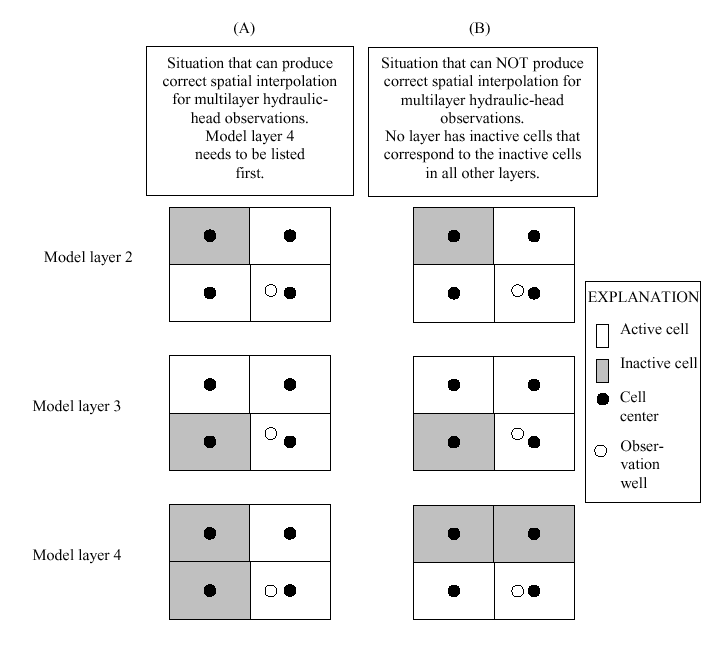Figure 4: Situations for which the Observation Process (A) can and (B) cannot produce correct spatial interpolation for the multilayer hydraulic-head observation shown in figure 3.ROW
 ROW—is the row index of the cell in which the head observation is located.COLUMN
 COLUMN—is the column index of the cell in which the head observation is located.IREFSP
 IREFSP—is the stress period to which the observation time is referenced. The reference point is the beginning of the specified stress period. If the value of IREFSP read in item 3 is negative, there are observations at |IREFSP| times -- item 5 is read and |IREFSP| repetitions of item 6 are read. Also, if IREFSP is negative, values of OBSNAM, TOFFSET, HOBS, STATISTIC, STAT-FLAG and PLOT-SYMBOL read in item 3 are ignored and values read in item 6 are used.TOFFSET
 TOFFSET—is the time from the beginning of stress period IREFSP to the time of the observation [T]. TOFFSET must be in units such that the product of TOMULTH (in item 2 above) and TOFFSET is in time units consistent with other model input. TOFFSET and TOMULTH from the HOB file and values of PERLEN, NSTP, and TSMULT from the Discretization file (Harbaugh and others, 2000) are used to determine the stress period, time step, and time during the time step for the observation. To specify that an observation is for a steady-state model solution, specify IREFSP as the stress-period number of the steady-state stress period, and specify TOFFSET such that the product TOMULTH*TOFFSET is less than or equal to PERLEN for the stress period; if PERLEN is zero, set TOFFSET to zero. If the observation falls within a time step, the simulated equivalent is calculated by linearly interpolating between heads at the beginning and end of the time step. If the first stress period is transient and the observation falls within the first time step of the stress period, the head from the beginning of the time step is determined by using the initial head distribution specified in the Basic Package input file.ROFF
 ROFF—is the row offset used to locate the observation within a finite-difference cell (fig. 2). If the absolute value of ROFF is less than 0.001, ROFF is changed to 0.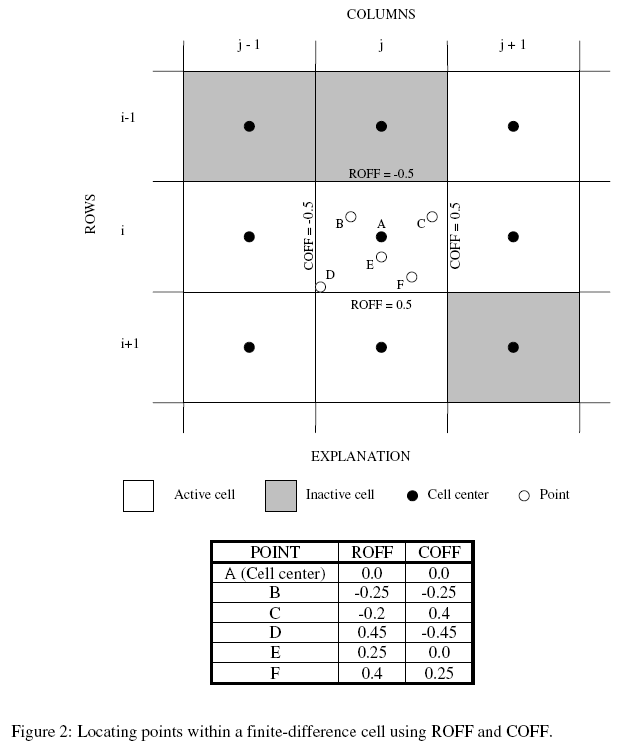COFF
 COFF—is the column offset used to locate the observation within a finite-difference cell (fig. 2). If the absolute value of COFF is less than 0.001, COFF is changed to 0.HOBS
 HOBS—is the observed hydraulic head [L]. In item 6, this needs to be hydraulic head even when ITT=2 in item 5; the program will perform the required subtraction.STATISTIC
 STATISTIC—is the value from which the observation weight is calculated as determined using STAT-FLAG.STAT-FLAG

STAT-FLAG—is a flag identifying what STATISTIC is and how the observation weight is calculated.

 • STAT-FLAG = 0, STATISTIC is a scaled variance [L2], weight = 1/(STATISTIC * EVH),
 • STAT-FLAG = 1, STATISTIC is a scaled standard deviation [L], weight = 1/(STATISTIC2 * EVH), and
 • STAT-FLAG = 2, STATISTIC is a scaled coefficient of variation [--], weight = 1/[(STATISTIC * HOBS)2 * EVH].PLOT-SYMBOL
 PLOT-SYMBOL—is an integer that is written to output files intended for graphical analysis to allow control of the symbols used to plot data.

Data Set 4

MLAY(1), PR(1), MLAY(2), PR(2), ..., MLAY(|LAYER|), PR(|LAYER|) (free format)

Sufficient repetitions of item 4 are read to define the contributions from each layer. The order of the layers needs to be specified according to the method presented in figure 4.Explanation of variablesMLAY
 MLAY(I)—is the I'th layer number for a multilayer head observation.PR
 PR(I)—is the proportion of the simulated hydraulic head in layer MLAY(I) that is used to calculate simulated multilayer head. The sum of all PR values for a given observation needs to equal 1.0.Figure 4Figure 4: Situations for which the Observation Process (A) can and (B) cannot produce correct spatial interpolation for the multilayer hydraulic-head observation shown in figure 3.

Data Set 5

ITT (free format)

If IREFSP in item 3 is less than zero, read item 5.ITT

ITT—is a flag that identifies whether head or changes in head are to be used as observations.

 • ITT = 1: The observed hydraulic heads are used as observations.
 • ITT = 2: The initial observed hydraulic head and subsequent changes in head (for example, drawdown) are used as observations. Changes in head are calculated internally from the hydraulic-head values listed in item 6, so the HOBS values specified in item 6 need to be hydraulic heads.

Data Set 6

 MODFLOW-2000: OBSNAM IREFSP TOFFSET HOBS STATh STATdd  STAT-FLAG PLOT-SYMBOL (free format) MODFLOW-2005, MODFLOW-CFP, MODFLOW-NWT, MODFLOW-OWHM, and MODFLOW-LGR: OBSNAM IREFSP TOFFSET HOBS (free format)

If IREFSP in item 3 is less than zero, read item 6 for each of IREFSP observation timesExplanation of variablesOBSNAM
 OBSNAM—is a string of 1 to 12 nonblank characters used to identify the observation. The identifier need not be unique; however, identification of observations in the output files is facilitated if each observation is given a unique OBSNAM.  The current version of MODFLOW-2000 (v 1.17.01) accepts duplicate observation names, but if duplicate names are found, a warning concerning the duplication is written to the Global file.IREFSP
 IREFSP—is the stress period to which the observation time is referenced. The reference point is the beginning of the specified stress period. If the value of IREFSP read in item 3 is negative, there are observations at |IREFSP| times -- item 5 is read and |IREFSP| repetitions of item 6 are read. Also, if IREFSP is negative, values of OBSNAM, TOFFSET, HOBS, STATISTIC, STAT-FLAG and PLOT-SYMBOL read in item 3 are ignored and values read in item 6 are used.TOFFSET
 TOFFSET—is the time from the beginning of stress period IREFSP to the time of the observation [T]. TOFFSET must be in units such that the product of TOMULTH (in item 2 above) and TOFFSET is in time units consistent with other model input. TOFFSET and TOMULTH from the HOB file and values of PERLEN, NSTP, and TSMULT from the Discretization file (Harbaugh and others, 2000) are used to determine the stress period, time step, and time during the time step for the observation. To specify that an observation is for a steady-state model solution, specify IREFSP as the stress-period number of the steady-state stress period, and specify TOFFSET such that the product TOMULTH*TOFFSET is less than or equal to PERLEN for the stress period; if PERLEN is zero, set TOFFSET to zero. If the observation falls within a time step, the simulated equivalent is calculated by linearly interpolating between heads at the beginning and end of the time step. If the first stress period is transient and the observation falls within the first time step of the stress period, the head from the beginning of the time step is determined by using the initial head distribution specified in the Basic Package input file.HOBS
 HOBS—is the observed hydraulic head [L]. In item 6, this needs to be hydraulic head even when ITT=2 in item 5; the program will perform the required subtraction.STATh
 STATh—is the value from which the weight is calculated if the observation is hydraulic head.  STAT-FLAG is used to identify what STATh is and how the weight is calculated, as for STATISTIC.STATdd
 STATdd—is the value from which the weight is calculated if the observation is the temporal change in hydraulic head. STAT-FLAG is used to identify what STATdd is and how the weight is calculated, as for STATISTIC.STAT-FLAG

STAT-FLAG—is a flag identifying what STATISTIC is and how the observation weight is calculated.

 • STAT-FLAG = 0, STATISTIC is a scaled variance [L2], weight = 1/(STATISTIC * EVH),
 • STAT-FLAG = 1, STATISTIC is a scaled standard deviation [L], weight = 1/(STATISTIC2 * EVH), and
 • STAT-FLAG = 2, STATISTIC is a scaled coefficient of variation [--], weight = 1/[(STATISTIC * HOBS)2 * EVH].PLOT-SYMBOL
 PLOT-SYMBOL—is an integer that is written to output files intended for graphical analysis to allow control of the symbols used to plot data.